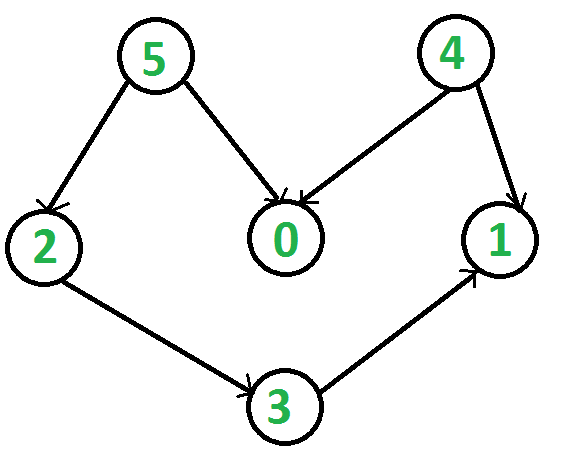# C++ Program for Topological Sorting

Topological sorting for Directed Acyclic Graph (DAG) is a linear ordering of vertices such that for every directed edge uv, vertex u comes before v in the ordering. Topological Sorting for a graph is not possible if the graph is not a DAG.

For example, a topological sorting of the following graph is “5 4 2 3 1 0”. There can be more than one topological sorting for a graph. For example, another topological sorting of the following graph is “4 5 2 3 1 0”. The first vertex in topological sorting is always a vertex with in-degree as 0 (a vertex with no in-coming edges).`// A C++ program to print topological sorting of a DAG ` `#include ` `#include ` `#include ` `using` `namespace` `std; ` ` `  `// Class to represent a graph ` `class` `Graph { ` `    ``int` `V; ``// No. of vertices' ` ` `  `    ``// Pointer to an array containing adjacency listsList ` `    ``list<``int``>* adj; ` ` `  `    ``// A function used by topologicalSort ` `    ``void` `topologicalSortUtil(``int` `v, ``bool` `visited[], stack<``int``>& Stack); ` ` `  `public``: ` `    ``Graph(``int` `V); ``// Constructor ` ` `  `    ``// function to add an edge to graph ` `    ``void` `addEdge(``int` `v, ``int` `w); ` ` `  `    ``// prints a Topological Sort of the complete graph ` `    ``void` `topologicalSort(); ` `}; ` ` `  `Graph::Graph(``int` `V) ` `{ ` `    ``this``->V = V; ` `    ``adj = ``new` `list<``int``>[V]; ` `} ` ` `  `void` `Graph::addEdge(``int` `v, ``int` `w) ` `{ ` `    ``adj[v].push_back(w); ``// Add w to v’s list. ` `} ` ` `  `// A recursive function used by topologicalSort ` `void` `Graph::topologicalSortUtil(``int` `v, ``bool` `visited[], ` `                                ``stack<``int``>& Stack) ` `{ ` `    ``// Mark the current node as visited. ` `    ``visited[v] = ``true``; ` ` `  `    ``// Recur for all the vertices adjacent to this vertex ` `    ``list<``int``>::iterator i; ` `    ``for` `(i = adj[v].begin(); i != adj[v].end(); ++i) ` `        ``if` `(!visited[*i]) ` `            ``topologicalSortUtil(*i, visited, Stack); ` ` `  `    ``// Push current vertex to stack which stores result ` `    ``Stack.push(v); ` `} ` ` `  `// The function to do Topological Sort. It uses recursive ` `// topologicalSortUtil() ` `void` `Graph::topologicalSort() ` `{ ` `    ``stack<``int``> Stack; ` ` `  `    ``// Mark all the vertices as not visited ` `    ``bool``* visited = ``new` `bool``[V]; ` `    ``for` `(``int` `i = 0; i < V; i++) ` `        ``visited[i] = ``false``; ` ` `  `    ``// Call the recursive helper function to store Topological ` `    ``// Sort starting from all vertices one by one ` `    ``for` `(``int` `i = 0; i < V; i++) ` `        ``if` `(visited[i] == ``false``) ` `            ``topologicalSortUtil(i, visited, Stack); ` ` `  `    ``// Print contents of stack ` `    ``while` `(Stack.empty() == ``false``) { ` `        ``cout << Stack.top() << ``" "``; ` `        ``Stack.pop(); ` `    ``} ` `} ` ` `  `// Driver program to test above functions ` `int` `main() ` `{ ` `    ``// Create a graph given in the above diagram ` `    ``Graph g(6); ` `    ``g.addEdge(5, 2); ` `    ``g.addEdge(5, 0); ` `    ``g.addEdge(4, 0); ` `    ``g.addEdge(4, 1); ` `    ``g.addEdge(2, 3); ` `    ``g.addEdge(3, 1); ` ` `  `    ``cout << ``"Following is a Topological Sort of the given graph n"``; ` `    ``g.topologicalSort(); ` ` `  `    ``return` `0; ` `} `

Output:

```Following is a Topological Sort of the given graph n5 4 2 3 1 0
```

Please refer complete article on Topological Sorting for more details!

My Personal Notes arrow_drop_up

Article Tags :

Be the First to upvote.

Please write to us at contribute@geeksforgeeks.org to report any issue with the above content.